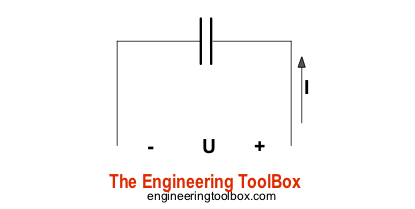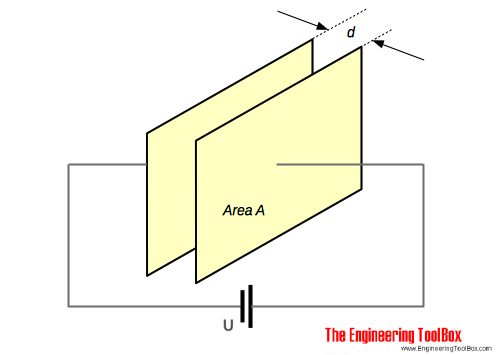Engineering ToolBox - Resources, Tools and Basic Information for Engineering and Design of Technical Applications!

# Capacitors

## Capacitors and capacitance - charge and unit of charge.

A capacitor is a device used to store electrical energy.The plates of a capacitor is charged and there is an electric field between them. The capacitor will be discharged if the plates are connected together through a resistor.

### Charge of a Capacitor

The charge of a capacitor can be expressed as

Q = I t                   (1)

where

Q = charge of capacitor (coulomb, C, mC)

I = current (amp, A)

t = time (s)

The quantity of charge (number of electrons) is measured in the unit Coulomb - C - where

1 coulomb = 6.24 1018 electrons

The smallest charge that exists is the charge carried by an electron, equal to -1.602 10-19 coulomb.

#### Example - Quantity of Electricity Transferred

If a current of 5 amp flows for 2 minutes, the quantity of electricity - coulombs - can be calculated as

Q = (5 A) (2 min) (60 s/min)

= 600

or, in electrons:

(600 C) (6.24 1018 electrons / C)

= 3.744 1021 electrons

### Electric Field Strength (Dielectric Strength)If two charged plates are separated with an insulating medium - a dielectric -  the electric field strength (potential gradient) between the two plates can be expressed as

E = U / d                        (2)

where

E = electric field strength (volts/m)

U = eletrical potential (volt)

d = thickness of dielectric, distance between plates (m)

#### Example - Electric Field Strength

The voltage between two plates is 230 V and the distance between them is 5 mm. The electric field strength can be calculated as

E = (230 V) / ((5 mm) (10-3 m/mm))

= 46000 volts/m

= 46 kV/m

### Electric Flux Density

Electric flux density is the ratio between the charge of the capacitor and the surface area of the capacitor plates:

D = Q / A                       (3)

where

D = electric flux density (coulomb/m2)

A = surface area of the capacitor (m2)

### Charge and Applied Voltage

Charge in a capacitor is proportional to the applied voltage and can be expressed as

Q = C U                        (4)

where

C = constant of proportionality or capacitance (farad, F, µF )

### Capacitance

From (4) the capacitance can be expressed as

C = Q / U                         (5)

One farad is defined as the capacitance of a capacitor when there is a potential difference across the plates of one volt when holding a charge of one coulomb.

It is common to use µF (10-6 F)

#### Example - Voltage over a Capacitor

A 5 µF capacitor is charged with 10 mC. The voltage across the capacitor can be calculated by modifying (4) to

U = Q / C

= (10 mC) (10-3 C/mC) / ((5 µF) (10-6 F/µF)

= 2000 V

= 2 kV

### Absolute Permittivity

The ratio of electric flux density to electric field is called absolute permittivity - ε - of a dielectric and can be expressed as

ε = D / E                     (6)

where

ε = absolute permittivity (F/m)

The absolute permittivity of free space or vacuum - also called the electric constant - ε0 - is 8.85 10-12 F/m.

### Relative Permittivity

Relative permittivity - also called the dielectric constant  εr - is the ratio between the flux density of the field in an actual dielectric - ε - and the flux density of the field in absolute vacuum - ε0.

εr = ε / ε0                   (7a)

The actual permittivity can be calculated by transforming (7a) to

ε = εr ε0                      (7b)

### Parallel Plate Capacitor

The capacitance of a plate capacitor - as shown in the figure above - is proportional with the area A of the plate. The capacitance can be expressed as

C = εr ε0 A / d                   (8)

where

A = area of plate (m2)

d = thickness of dielectric, distance between plates (m)

For a plate capacitor with multiple plates the capacitance can be expressed as

C = εr ε0 A (n - 1) / d                      (8b)

where

n = number of plates

### Example - Capacitance of a Plate Capacitor

The capacitance of a plate capacitor with area 5 cm2, 10 plates and distance 0.1 mm between the plates - with ceramic dielectric with relative permittivity 30 between the plates - can be calculated as

C = (8.85 10-12 F/m) (30) (5 cm2) (10-4 m2/cm2) (10 - 1) / ((0.1 mm) (10-3 m/mm))

= 11 10-9 F

= 11 pF

### Typical commonly used Capacitors

Typical capacitors are

• variable air capacitors
• mica capacitors
• paper capacitors
• ceramic capacitors
• plastic capacitors
• titanium oxide capacitors
• electrolytic capacitors

## Related Topics

• Electrical - Electrical units, amps and electrical wiring, wire gauge and AWG, electrical formulas and motors.

## Engineering ToolBox - SketchUp Extension - Online 3D modeling!

Add standard and customized parametric components - like flange beams, lumbers, piping, stairs and more - to your Sketchup model with the Engineering ToolBox - SketchUp Extension - enabled for use with the amazing, fun and free SketchUp Make and SketchUp Pro .Add the Engineering ToolBox extension to your SketchUp from the SketchUp Pro Sketchup Extension Warehouse!

Translate

## Privacy

We don't collect information from our users. Only emails and answers are saved in our archive. Cookies are only used in the browser to improve user experience.

Some of our calculators and applications let you save application data to your local computer. These applications will - due to browser restrictions - send data between your browser and our server. We don't save this data.

## Citation

• Engineering ToolBox, (2008). Capacitors. [online] Available at: https://www.engineeringtoolbox.com/capacitors-d_1387.html [Accessed Day Mo. Year].

Modify access date.

. .

#### Scientific Online Calculator3 30